JEE  >  BITSAT Maths Test - 3

# BITSAT Maths Test - 3

Test Description

## 45 Questions MCQ Test BITSAT Mock Tests Series & Past Year Papers | BITSAT Maths Test - 3

BITSAT Maths Test - 3 for JEE 2023 is part of BITSAT Mock Tests Series & Past Year Papers preparation. The BITSAT Maths Test - 3 questions and answers have been prepared according to the JEE exam syllabus.The BITSAT Maths Test - 3 MCQs are made for JEE 2023 Exam. Find important definitions, questions, notes, meanings, examples, exercises, MCQs and online tests for BITSAT Maths Test - 3 below.
Solutions of BITSAT Maths Test - 3 questions in English are available as part of our BITSAT Mock Tests Series & Past Year Papers for JEE & BITSAT Maths Test - 3 solutions in Hindi for BITSAT Mock Tests Series & Past Year Papers course. Download more important topics, notes, lectures and mock test series for JEE Exam by signing up for free. Attempt BITSAT Maths Test - 3 | 45 questions in 60 minutes | Mock test for JEE preparation | Free important questions MCQ to study BITSAT Mock Tests Series & Past Year Papers for JEE Exam | Download free PDF with solutions
 1 Crore+ students have signed up on EduRev. Have you?
BITSAT Maths Test - 3 - Question 1

### The area common to the parabola y = 2x2 and y = x2 + 4 is

BITSAT Maths Test - 3 - Question 2

### 3nC₀-8nC₁+13nC₂-18nC₃+...+ to (n+1) terms=

BITSAT Maths Test - 3 - Question 3

### The solution of differential equation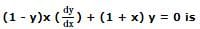Detailed Solution for BITSAT Maths Test - 3 - Question 3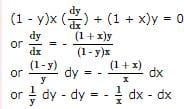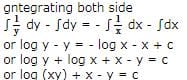BITSAT Maths Test - 3 - Question 4

The equation of the line parallel to the tangent to the circle x2 + y2 = r2 at the point (x₁, y₁) and passing thro' origin is

Detailed Solution for BITSAT Maths Test - 3 - Question 4

Explanation : mm1 = -1

(y1/x1)*m1 = -1

m1 = - x1/y1

So, equation of line passing through (x₁, y₁)

y = m1x

y = (-x1/y1)*x

yy1+ xx1 = 0

BITSAT Maths Test - 3 - Question 5

The lines 2x-3y=5 and 3x-4y=7 are diameters of a circle of area 154 sq. units. Then the equation of this circle is :

BITSAT Maths Test - 3 - Question 6

The solution of the equation cosx cosy (dy/dx)=-sinx siny is

BITSAT Maths Test - 3 - Question 7

The differential equation with respect to the curve y=emx is

BITSAT Maths Test - 3 - Question 8

The agrument of (1 - i√3)/(1 + √3) is

Detailed Solution for BITSAT Maths Test - 3 - Question 8

Solution :- ⇒z = [(1−i(3)^1/2)^2 * (1+i(3)^1/2)^2]/[(1+i(3)^1/2)^2 * (1−i(3)^1/2)^2]

⇒z = [(1−i(3)^1/2)^2​]/(1+3)

⇒z = [1−3−2i(3)^½]/4

⇒z = − 1/2 − [i(3)^½]/2

r = [(-½)^2 + (-(3)^½ /2)^2]^½    =1

Comparing the above equation with z=rcosα+irsinα

rcosα = − 1/2   ⇒cosα = −1/2

rsinα = − (3)^1/2/2      ⇒sinα = −(3)^1/2/2

Since sinα and cosα, both are negative, thus the argument will be in IIIrd

α=180°+60°=240°(∵sin60° = (3)^1/2/2 & cos60° = 1/2)

Hence argument of given complex is 240°.

BITSAT Maths Test - 3 - Question 9

(d/dx)[tan⁻1((sinx+cosx)/(cosx-sinx))]

BITSAT Maths Test - 3 - Question 10

The sum of the series 2/3! + 4/5! + 6/7! + ......∞ is

BITSAT Maths Test - 3 - Question 11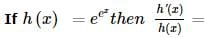BITSAT Maths Test - 3 - Question 12

At a point a metres high above a lake the angle of elevation of a cloud is α and the angle of depression of its image is β, the height of the cloud is

BITSAT Maths Test - 3 - Question 13

The tangents to the hyperbola x2 - y2 = 3 are parallel to the st. line 2x + y + 8 = 0 at the following points

BITSAT Maths Test - 3 - Question 14

The differential equation (d2y/dx2)2/3 = (y + (dy/dx))1/2 is of

Detailed Solution for BITSAT Maths Test - 3 - Question 14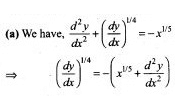Order = 2, Degree = 4

BITSAT Maths Test - 3 - Question 15

The solution of the differential equation (dy/dx) = (y/x) + (φ (y/x)/φ' (y/x)) is

BITSAT Maths Test - 3 - Question 16

The domain of sin⁻1 x is

BITSAT Maths Test - 3 - Question 17

The function f(x) = cot⁻1 x + x increases in the interval

BITSAT Maths Test - 3 - Question 18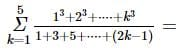BITSAT Maths Test - 3 - Question 19

For a skew symmetric odd ordered matrix A of integers, which of the following will hold true:

BITSAT Maths Test - 3 - Question 20

The sum of the products of the elements of any row of a determinant A with the same row is always equal to

BITSAT Maths Test - 3 - Question 21

The point on the curve y = x2 + 4x + 3 which is closest to the line y = 3x + 2 is

BITSAT Maths Test - 3 - Question 22

Which of the following statements are true ?
(1) The amplitude of the product of complex numbers is equal to the product of their amplitudes.
(2) For any polynomial f(x) =0 with real co-efficients, imaginary roots occurs in conjugate paris.
(3) Order relation exists in complex numbers whereas it does not exist in real numbers.
(4) The value of ω used as a cube root of unity and as a fourth root of unity are different.

BITSAT Maths Test - 3 - Question 23

The acute angle between the lines joining origin to the intersection points of the curve x2+y2-2x-1=0 and line x+y=1 is

BITSAT Maths Test - 3 - Question 24

The focus of the parabola x2 − 2 x − 8 y − 23 = 0 is

BITSAT Maths Test - 3 - Question 25

The focus of the parabola (y-2)2=20(x+3) is

BITSAT Maths Test - 3 - Question 26

The number of parallelograms that can be formed from a set of four parallel lines intersecting another set of there parallel lines is :

Detailed Solution for BITSAT Maths Test - 3 - Question 26

Solution :- To form parallelogram we required a pair of line from a set of 4 lines and another pair of line from another set of 3 lines.

Required number of parallelograms = 4C2 x 3C2 = 6×3 = 18

BITSAT Maths Test - 3 - Question 27

A group of 7 is to be formed from 6 boys and 4 girls. In many ways can this be done if the boys are in majority?

BITSAT Maths Test - 3 - Question 28

In eight throws of 1 die or 3 is considered a success. Then the standard deviation of the success is

BITSAT Maths Test - 3 - Question 29

In a competitions A, B and C are participating. The probability that A wins is twice that of B, the probability that B wins is twice that of C. The probability that A loses is

Detailed Solution for BITSAT Maths Test - 3 - Question 29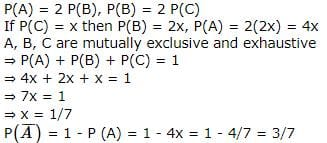BITSAT Maths Test - 3 - Question 30

In a triangle ABC, A = 30o, b = 8, a = 6, then B = sin⁻1 x where x =

BITSAT Maths Test - 3 - Question 31

In Δ A B C , if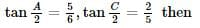BITSAT Maths Test - 3 - Question 32

x2+x+1+2k(x2-x-1)=0 is a perfect square for how many values of k?

BITSAT Maths Test - 3 - Question 33

If the arithmetic and geometric means of two distinct, positive numbers are A and G respectively, then their harmonic mean is

BITSAT Maths Test - 3 - Question 34

a, b, c are three unequal numbers such that a, b, c are in A.P. ; b - a, c - b, a are in G.P., then a : b : c : :

Detailed Solution for BITSAT Maths Test - 3 - Question 34

Since a, b, c are in A.P.,
∴ b - a = c - b
Also b - a, c - b, a are in G.P
∴ (c - b)2 = (b - a)a
⇒ (b - a)2 = (b - a)a
⇒ b - a = a
⇒ b = 2a
Also c = 2b - a = 2(2a) - a = 3a
∴ a : b : c = a : 2a : 3a = 1 : 2 : 3

BITSAT Maths Test - 3 - Question 35

If A and B are two sets such that n(A) = 70, n(B) = 60 and n(A ∪ B)= 110, then n (A ∩ B) is equal to

BITSAT Maths Test - 3 - Question 36

The minimum value of sin6x + cos6x is

Detailed Solution for BITSAT Maths Test - 3 - Question 36

Solution :- y= sin6(x) + cos6(x).

y =(sin2x)3 + (cos2x)3

y=(sin2x+cos2x)(sin4x+cos4x-sin2x.cos2x)

y=(1).[ (sin2x+cos2x)2–3.sin2x.cos2x]

y= 1 - 3 sin2x.cos2x

y = 1 -3(sin x.cos x)2

y= 1- 3(1/2.sin2x)2

y = 1 - (3/4).(sin 2x)2

For minimum value of y , sin2x should be maximum , maximum value of sin 2x =1 or

x=45°.

Minimum value of y= 1 -(3/4).(1)2

= 1 - 3/4 = 1/4

BITSAT Maths Test - 3 - Question 37

The equation of a line passing through the intersection of lines 3x-2y-1=0 and x-4y+3=0 and point (π,0) is

BITSAT Maths Test - 3 - Question 38

If y = 4x - 5 is a tangent to the curve y2 = ax3 + b at (2, 3), then

BITSAT Maths Test - 3 - Question 39

The smallest radius of the sphere passing thro'(1,0,0),(0,1,0) and (0,0,1) is

Detailed Solution for BITSAT Maths Test - 3 - Question 39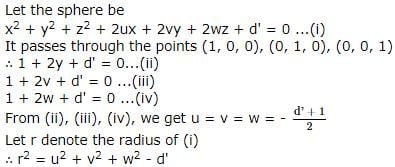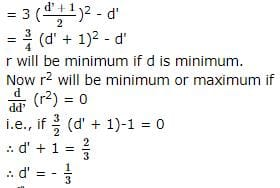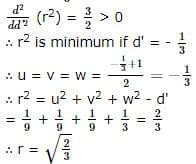BITSAT Maths Test - 3 - Question 40

The equation of the sphere concentric with the sphere x2+y2+z2-4x-6y-8z=0 and which passes thro' (0.1,0) is

BITSAT Maths Test - 3 - Question 41

If α is a root of 25cos2θ + 5cosθ - 12 = 0, (π/2)<α<π, then sin2α is equal to

BITSAT Maths Test - 3 - Question 42

If 0≤x≤π and 81sin2x + 81 cos2x = 30, then x is equal to

BITSAT Maths Test - 3 - Question 43

Given that θ is a acute and that sin θ =3/5. Let x,y be positive real numbers such that 3(x-y) =1, then one set of solutions for x and y expressed in terms of θ is given by

BITSAT Maths Test - 3 - Question 44

The number of vectors of unit length perpendicular to vectors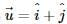and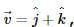is

BITSAT Maths Test - 3 - Question 45

A force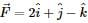acts at a point A whose position vector is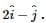If point of application of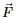moves from A to the point B with position vector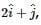then work done byis

## BITSAT Mock Tests Series & Past Year Papers

2 videos|15 docs|70 tests
 Use Code STAYHOME200 and get INR 200 additional OFF Use Coupon Code
Information about BITSAT Maths Test - 3 Page
In this test you can find the Exam questions for BITSAT Maths Test - 3 solved & explained in the simplest way possible. Besides giving Questions and answers for BITSAT Maths Test - 3, EduRev gives you an ample number of Online tests for practice

## BITSAT Mock Tests Series & Past Year Papers

2 videos|15 docs|70 tests